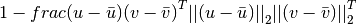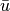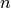# scipy.spatial.distance.correlation¶

scipy.spatial.distance.correlation(u, v)

Computes the correlation distance between two n-vectors u and v, which is defined aswhereis the mean of a vectors elements and n is the common dimensionality of u and v.

Parameters : u : ndarray An-dimensional vector. v : ndarray An-dimensional vector. d : double The correlation distance between vectors u and v.

#### Previous topic

scipy.spatial.distance.cityblock

#### Next topic

scipy.spatial.distance.cosine